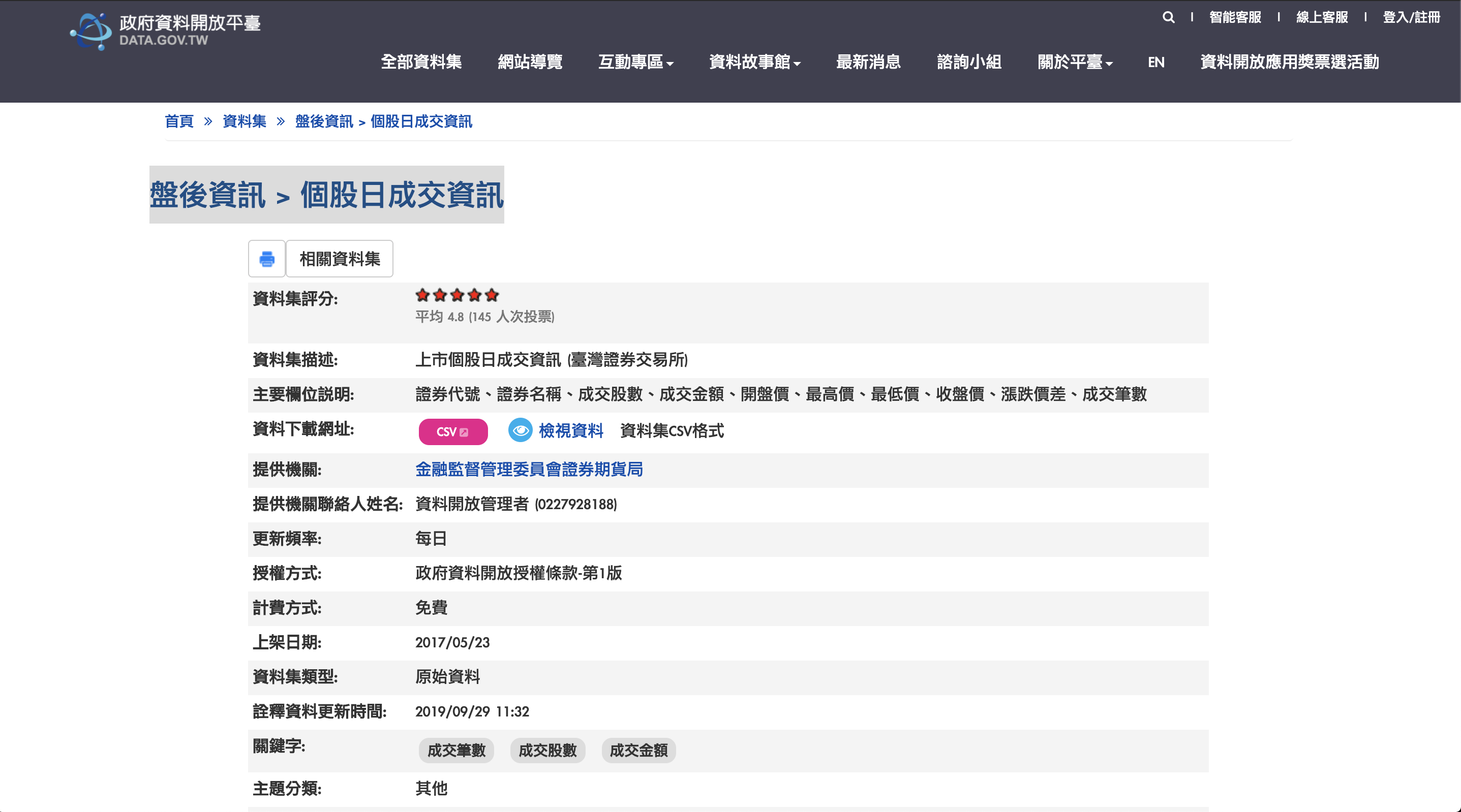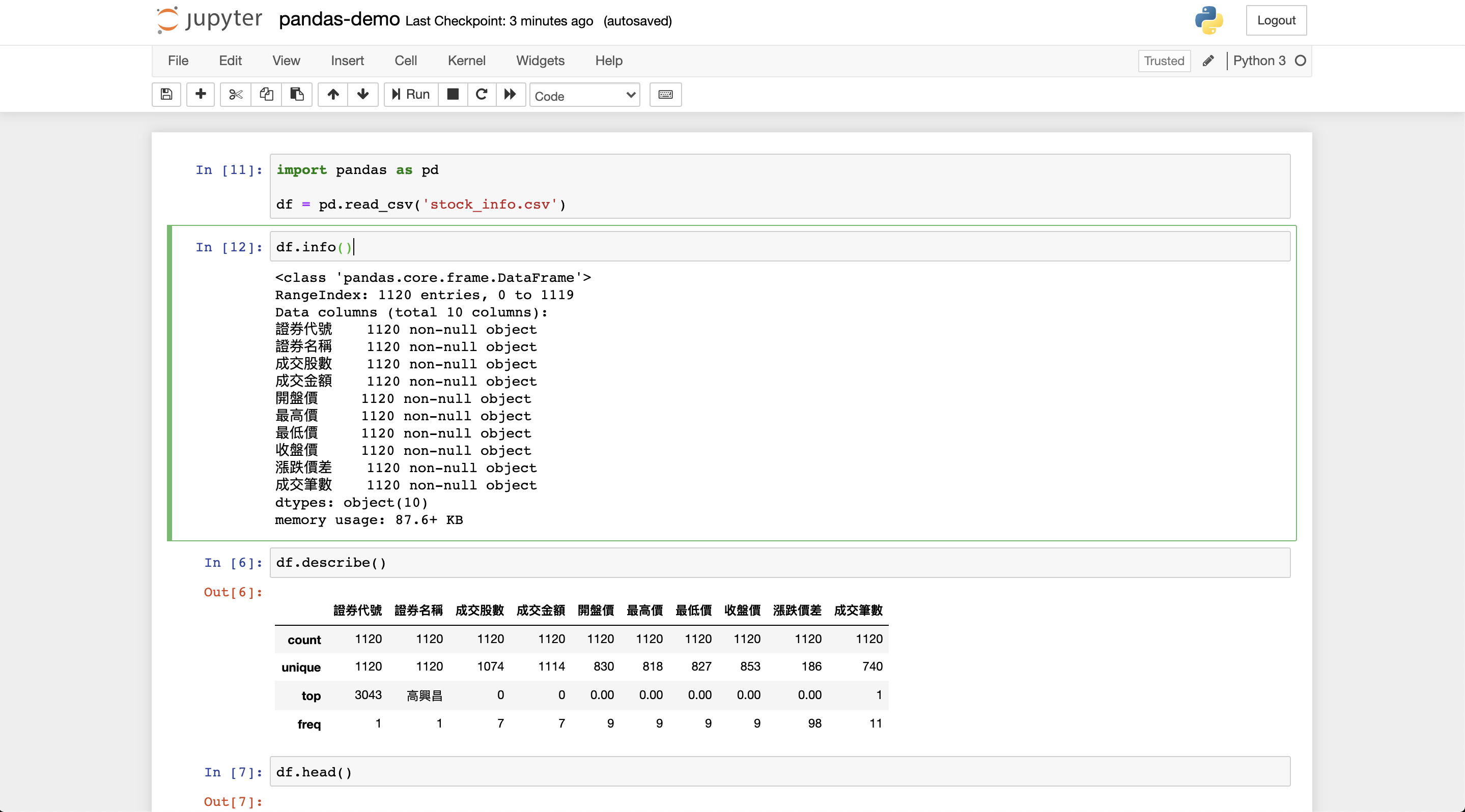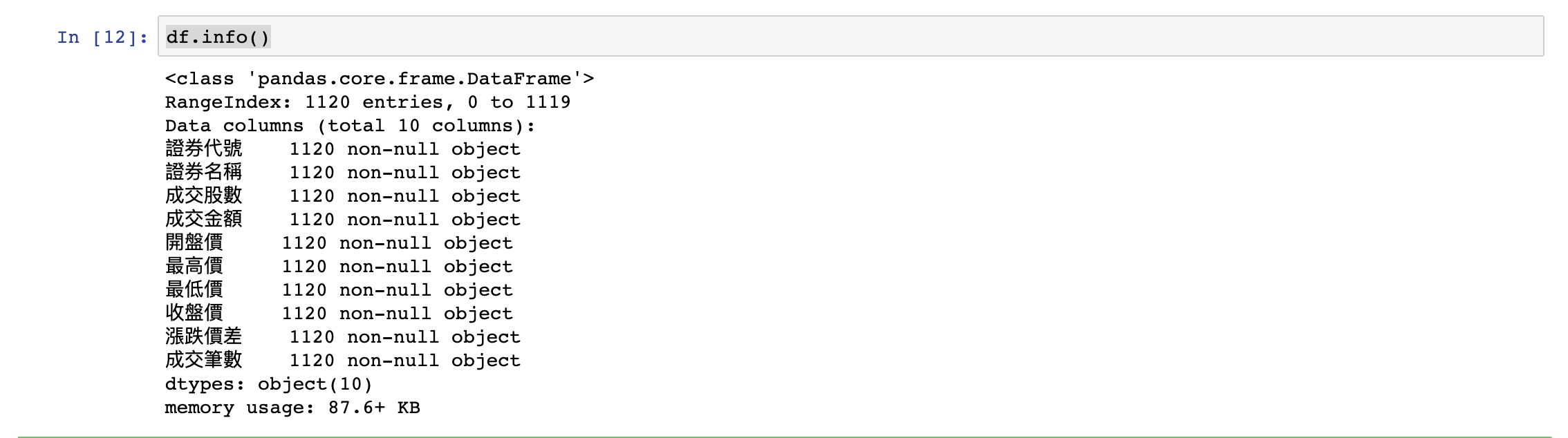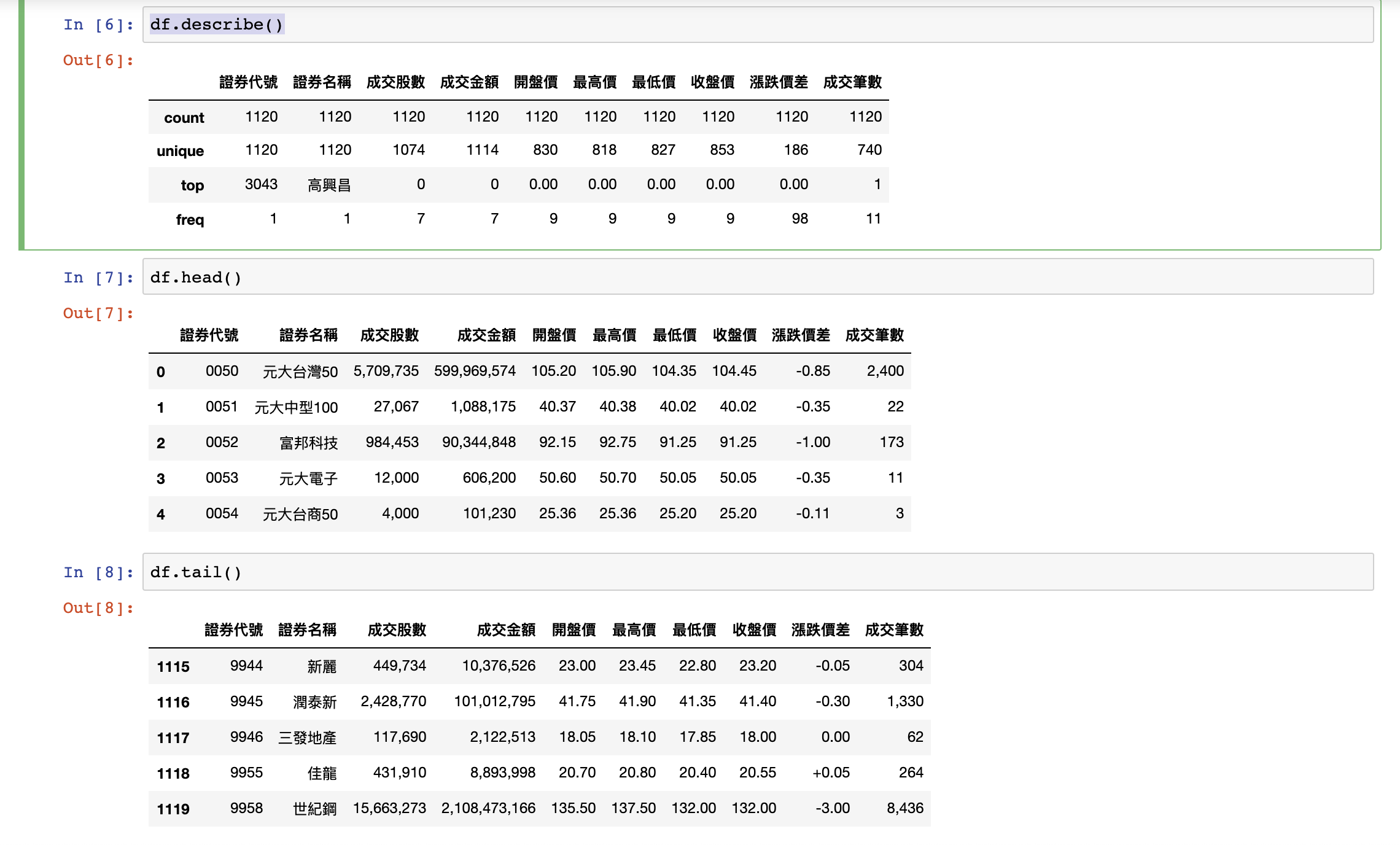# Pandas 套件基礎

Pandas 是 Python 進行資料處理和資料分析一個好用的工具，其主要資料結構有包含：Series 物件和 DataFrame 物件。其中 DataFrame 就類似我們在使用的 Excel 試算表一樣，由欄列所組成的表格結構。由於 Pandas 本身基於 Numpy 所以在使用大量資料運算時效能表現也優於原生的 Python 資料結構，所以是常用將資料載入進行資料分析的好用工具。

1. Series 物件 Series 為索引標籤和實際值的陣列組合
2. DataFrame 物件 DataFrame 類似試算表和關聯式資料庫資料表（table）欄列結構，每一欄是固定資料型別但不同欄可以儲存不同的資料型別

pip install pandas


!pip install pandas


## 建立 Series 物件

# 引入 pandas 套件，使用別名 pd 可以少打字
import pandas as pd

# 建立 Series 物件，傳入 list 當作參數
series_1 = pd.Series([2, 1, 7, 3])

print(series_1)


0    2
1    1
2    7
3    3
dtype: int64


# 引入 pandas 套件，使用別名 pd 可以少打字
import pandas as pd

# 建立 Series 物件並設定 index 索引
grades = pd.Series([60, 27, 72, 53], index=['小郭', '小王', '小華', '小明'])

print(grades)


小郭    60

dtype: int64


# 引入 pandas 套件，使用別名 pd 可以少打字
import pandas as pd

# 建立 Series 物件，傳入 list 當作參數
series_1 = pd.Series([22, 34, 41, 3])

print(series_1)
print(series_1[1:3])


12
1    34
2    41
dtype: int64


# 引入 pandas 套件，使用別名 pd 可以少打字
import pandas as pd

# 建立 Series 物件並設定 index 索引
grades = pd.Series([60, 27, 72, 53], index=['小郭', '小王', '小華', '小明'])

print(grades['小王'])


27


## 建立 DataFrame 物件

DataFramePandas 最重要的資料結構，基本上我們使用 Pandas 進行資料分析和操作大部分都是在使用 DataFrame。如同我們所提到的 DataFrame 的結構類似於關聯式資料庫的資料表（table）是由欄（column）和列的索引（index）所組成。

# 引入 pandas 套件，使用別名 pd 可以少打字
import pandas as pd

# 準備傳入
data = {
'name': ['王小郭', '張小華', '廖丁丁', '丁小光'],
'email': ['min@gmail.com', 'hchang@gmail.com', 'laioding@gmail.com', 'hsulight@gmail.com'],
'grades': [60, 77, 92, 43]
}

# 建立 DataFrame 物件
student_df = pd.DataFrame(data)

print(student_df)


  name               email  grades
0  王小郭       min@gmail.com      60
1  張小華    hchang@gmail.com      77
2  廖丁丁  laioding@gmail.com      92
3  丁小光  hsulight@gmail.com      43


# 引入 pandas 套件，使用別名 pd 可以少打字
import pandas as pd

# 準備傳入 DataFrame 的資料
data = {
'name': ['王小郭', '張小華', '廖丁丁', '丁小光'],
'email': ['min@gmail.com', 'hchang@gmail.com', 'laioding@gmail.com', 'hsulight@gmail.com'],
'grades': [60, 77, 92, 43]
}

# 建立 DataFrame 物件
student_df = pd.DataFrame(data, index=['one', 'second', 'third','fourth'])

print(student_df)


       name               email  grades
one     王小郭       min@gmail.com      60
second  張小華    hchang@gmail.com      77
third   廖丁丁  laioding@gmail.com      92
fourth  丁小光  hsulight@gmail.com      43


## 列出資料訊息

# 引入 pandas 套件，使用別名 pd 可以少打字
import pandas as pd

data = {
'name': ['王小郭', '張小華', '廖丁丁', '丁小光'],
'email': ['min@gmail.com', 'hchang@gmail.com', 'laioding@gmail.com', 'hsulight@gmail.com'],
'grades': [60, 77, 92, 43]
}

# 建立 DataFrame 物件
student_df = pd.DataFrame(data)

# 列出欄位資料型別等資訊
print(student_df.info())
# 列出統計資訊
print(student_df.describe())


<class 'pandas.core.frame.DataFrame'>
RangeIndex: 4 entries, 0 to 3
Data columns (total 3 columns):
name      4 non-null object
email     4 non-null object
grades    4 non-null int64
dtypes: int64(1), object(2)
memory usage: 176.0+ bytes
None
grades
count   4.000000
mean   68.000000
std    21.181753
min    43.000000
25%    55.750000
50%    68.500000
75%    80.750000
max    92.000000


# 列出 DataFrame 的 index/columns
print(student_df.index)
print(student_df.columns)


RangeIndex(start=0, stop=4, step=1)
Index(['name', 'email', 'grades'], dtype='object')


# 印出頭尾指定幾筆資料
print(student_df.head(3))
print(student_df.tail(3))


  name             email  grades
0  王小郭     min@gmail.com      60
1  張小華  hchang@gmail.com      77
2  廖丁丁  laioding@gmail.com    92
name               email  grades
1  張小華  hchang@gmail.com        77
2  廖丁丁  laioding@gmail.com      92
3  丁小光  hsulight@gmail.com      43


# Pandas DataFrame 基本操作

## 讀取資料Step1. 下載政府開放資料檔案

證券代號,證券名稱,成交股數,成交金額,開盤價,最高價,最低價,收盤價,漲跌價差,成交筆數
"0050","元大台灣50","5,709,735","599,969,574","105.20","105.90","104.35","104.45","-0.85","2,400"
"0051","元大中型100","27,067","1,088,175","40.37","40.38","40.02","40.02","-0.35","22"
"0052","富邦科技","984,453","90,344,848","92.15","92.75","91.25","91.25","-1.00","173"
"0053","元大電子","12,000","606,200","50.60","50.70","50.05","50.05","-0.35","11"
...


Step2. 載入資料

import pandas as pd

# 將 csv 檔案轉換成 DataFrame
df = pd.read_csv('stock_info.csv')Step3. 輸出資料狀況

# 輸入資料概況
df.info()
df.describe()

# 輸出頭尾資料
df.head()
df.tail()## 刪除欄位

# 引入 pandas 套件，使用別名 pd 可以少打字
import pandas as pd

# 準備傳入 DataFrame 的資料
data_1 = {
'name': ['王小郭', '張小華', '廖丁丁', '丁小光'],
'email': ['min@gmail.com', 'hchang@gmail.com', 'laioding@gmail.com', 'hsulight@gmail.com'],
'grades': [60, 77, 92, 43]
}

# 建立 DataFrame 物件
student_df_1 = pd.DataFrame(data_1)
print(student_df_1)

# 使用 drop 指定欄位，記得要給定 axis=1 為欄。若 axis=0 為代表列
student_df_1 = student_df_1.drop(['grades'], axis=1)
print(student_df_1)


  name               email  grades
0  王小郭       min@gmail.com      60
1  張小華    hchang@gmail.com      77
2  廖丁丁  laioding@gmail.com      92
3  丁小光  hsulight@gmail.com      43
name               email
0  王小郭       min@gmail.com
1  張小華    hchang@gmail.com
2  廖丁丁  laioding@gmail.com
3  丁小光  hsulight@gmail.com


## 充填 NA/NaN 值

# 引入 pandas 套件，使用別名 pd 可以少打字
import pandas as pd
import numpy as np

df = pd.DataFrame([[np.nan, 2, np.nan, 0],
[3, 4, np.nan, 1],
[np.nan, np.nan, np.nan, 5],
[np.nan, 3, np.nan, 4]],
columns=list('ABCD'))


df
A    B   C  D
0  NaN  2.0 NaN  0
1  3.0  4.0 NaN  1
2  NaN  NaN NaN  5
3  NaN  3.0 NaN  4


df.fillna(0)


    A   B   C   D
0   0.0 2.0 0.0 0
1   3.0 4.0 0.0 1
2   0.0 0.0 0.0 5
3   0.0 3.0 0.0 4


## 合併與更新物件

# 引入 pandas 套件，使用別名 pd 可以少打字
import pandas as pd

# 準備傳入 DataFrame 的資料
data_1 = {
'name': ['王小郭', '張小華', '廖丁丁', '丁小光'],
'email': ['min@gmail.com', 'hchang@gmail.com', 'laioding@gmail.com', 'hsulight@gmail.com'],
'grades': [60, 77, 92, 43]
}

data_2 = {
'name': ['黃明明', '汪新新', '鮑呱呱', '江組組'],
'email': ['ww@gmail.com', 'cc@gmail.com', 'bb@gmail.com', 'ee@gmail.com'],
'grades': [70, 17, 32, 43]
}

# 建立 DataFrame 物件
student_df_1 = pd.DataFrame(data_1)
student_df_2 = pd.DataFrame(data_2)

print(student_df_1)
print(student_df_2)


  name               email  grades
0  王小郭       min@gmail.com      60
1  張小華    hchang@gmail.com      77
2  廖丁丁  laioding@gmail.com      92
3  丁小光  hsulight@gmail.com      43
name         email  grades
0  黃明明  ww@gmail.com      70
1  汪新新  cc@gmail.com      17
2  鮑呱呱  bb@gmail.com      32
3  江組組  ee@gmail.com      43


student_fg_3 = pd.concat([student_df_1, student_df_2])

print(student_fg_3)


  name               email  grades
0  王小郭       min@gmail.com      60
1  張小華    hchang@gmail.com      77
2  廖丁丁  laioding@gmail.com      92
3  丁小光  hsulight@gmail.com      43
0  黃明明        ww@gmail.com      70
1  汪新新        cc@gmail.com      17
2  鮑呱呱        bb@gmail.com      32
3  江組組        ee@gmail.com      43


student_fg_3 = pd.concat([student_df_1, student_df_2], ignore_index=True)

print(student_fg_3)


  name               email  grades
0  王小郭       min@gmail.com      60
1  張小華    hchang@gmail.com      77
2  廖丁丁  laioding@gmail.com      92
3  丁小光  hsulight@gmail.com      43
4  黃明明        ww@gmail.com      70
5  汪新新        cc@gmail.com      17
6  鮑呱呱        bb@gmail.com      32
7  江組組        ee@gmail.com      43


# 引入 pandas 套件，使用別名 pd 可以少打字
import pandas as pd

# 準備傳入 DataFrame 的資料
data_1 = {
'name': ['王小郭', '張小華', '廖丁丁', '丁小光'],
'email': ['min@gmail.com', 'hchang@gmail.com', 'laioding@gmail.com', 'hsulight@gmail.com'],
'grades': [60, 77, 92, 43]
}

data_2 = {
'name': ['王小郭', '張小華', '廖丁丁', '丁小光'],
'age': [19, 20, 32, 43]
}

# 建立 DataFrame 物件
student_df_1 = pd.DataFrame(data_1)
student_df_2 = pd.DataFrame(data_2)

print(student_df_1)
print(student_df_2)


  name               email  grades
0  王小郭       min@gmail.com      60
1  張小華    hchang@gmail.com      77
2  廖丁丁  laioding@gmail.com      92
3  丁小光  hsulight@gmail.com      43
name  age
0  王小郭   19
1  張小華   20
2  廖丁丁   32
3  丁小光   43


student_fg_3 = pd.merge(student_df_1, student_df_2)

print(student_fg_3)


  name               email  grades  age
0  王小郭       min@gmail.com      60   19
1  張小華    hchang@gmail.com      77   20
2  廖丁丁  laioding@gmail.com      92   32
3  丁小光  hsulight@gmail.com      43   43


## 輸出資料

# 引入 pandas 套件，使用別名 pd 可以少打字
import pandas as pd

data = {
'name': ['王小郭', '張小華', '廖丁丁', '丁小光'],
'email': ['min@gmail.com', 'hchang@gmail.com', 'laioding@gmail.com', 'hsulight@gmail.com'],
'grades': [60, 77, 92, 43]
}

# 建立 DataFrame 物件
student_df = pd.DataFrame(data)

# 將 DataFrame 轉成 CSV 檔案
print(student_df.to_csv('student_demo.csv'))


,name,email,grades
0,王小郭,min@gmail.com,60
1,張小華,hchang@gmail.com,77
2,廖丁丁,laioding@gmail.com,92
3,丁小光,hsulight@gmail.com,43